## Coulomb Wave Function

A special case of the Confluent Hypergeometric Function of the First Kind. It gives the solution to the radial Schrödinger equationin the Coulomb potential(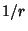) of a point nucleus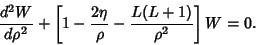(1)

The complete solution is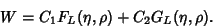(2)

The Coulomb function of the first kind is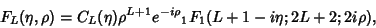(3)

where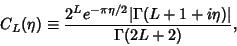(4)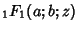is the Confluent Hypergeometric Function,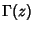is the Gamma Function, and the Coulomb function of the second kind is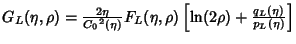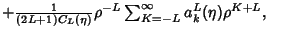(5)
where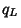,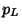, and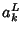are defined in Abramowitz and Stegun (1972, p. 538).

References

Abramowitz, M. and Stegun, C. A. (Eds.). Coulomb Wave Functions.'' Ch. 14 in Handbook of Mathematical Functions with Formulas, Graphs, and Mathematical Tables, 9th printing. New York: Dover, pp. 537-544, 1972.

Morse, P. M. and Feshbach, H. Methods of Theoretical Physics, Part I. New York: McGraw-Hill, pp. 631-633, 1953.